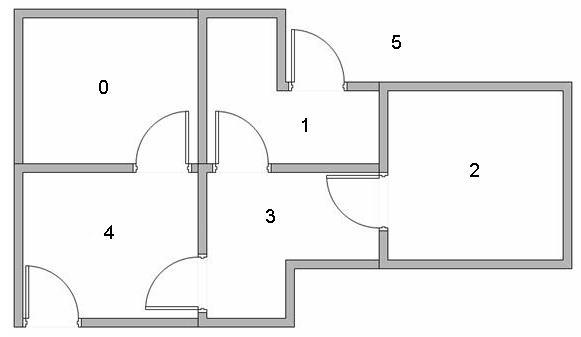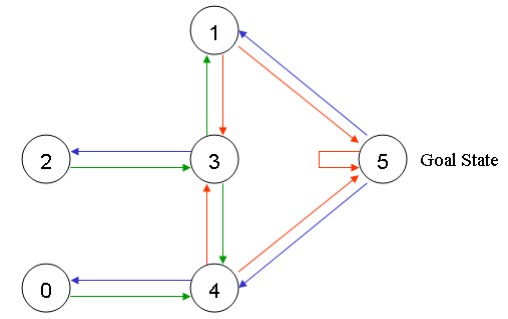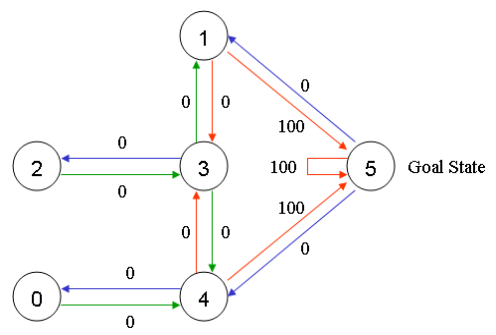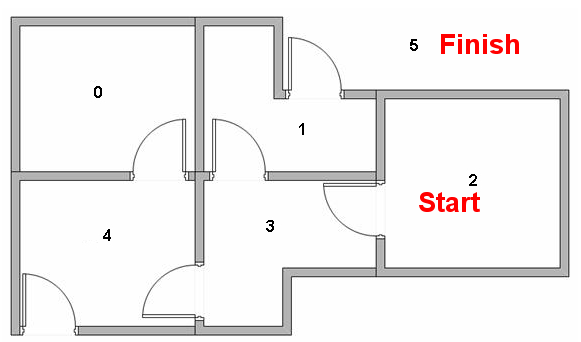Q-学习中的术语包括状态（state）和动作（action）。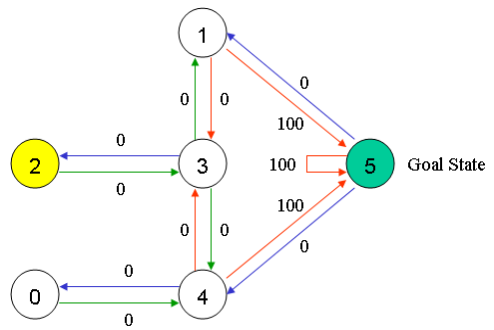（表中的-1代表空值，不允许通过）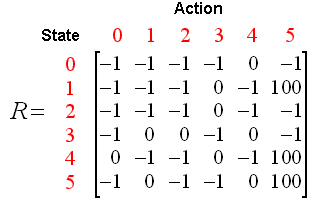Q-学习的转换规则非常简单，为下面的式子：

Q(state, action)=R(state, action) + Gamma * Max(Q[next state, all actions])

***********************************************************

PS：实际上，我在一些教材上，或者别的博客看到的公式大多都是下面这样的：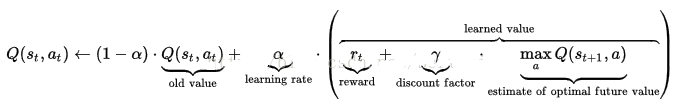***********************************************************

Q-Learning算法的计算过程如下：

1、设置参数Gamma，以及矩阵R中的环境奖励值；

2、初始化Q矩阵为0；

3、对每一次经历：

Do while 目标状态未到达

End For

************************************************************

PS：除此之外，在上一个补充说明部分的公式中，参数α也有类似的意义，不过α是对历史奖励的权衡，γ是对未来奖励的权衡。

************************************************************

1、设置当前状态=初始状态；

2、从当前状态开始，寻找具有最高Q值的动作；

3、设置当前状态=下一个状态；

4、重复步骤2和3，直到当前状态=目标状态。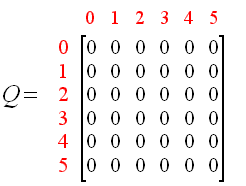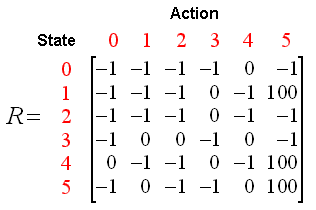Q(1, 5) = R(1, 5) + 0.8 * Max[Q(5, 1), Q(5, 4), Q(5, 5)] = 100 + 0.8 * 0 = 100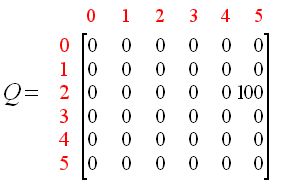PS：原作者的这个图有点错误，100应该是在Q(1, 5)的位置，而不是Q(2, 5)。

Q(3, 1) = R(3, 1) + 0.8 * Max[Q(1, 2), Q(1, 5)] = 0 + 0.8 * Max(0, 100) = 80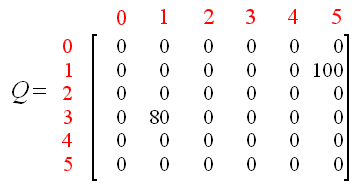Q(1, 5) = R(1, 5) + 0.8 * Max[Q(5, 1), Q(5, 4), Q(5, 5)] = 100 + 0.8 * 0 = 100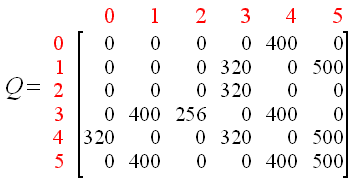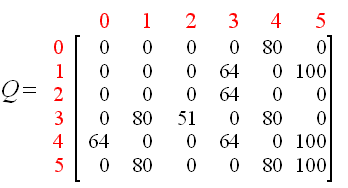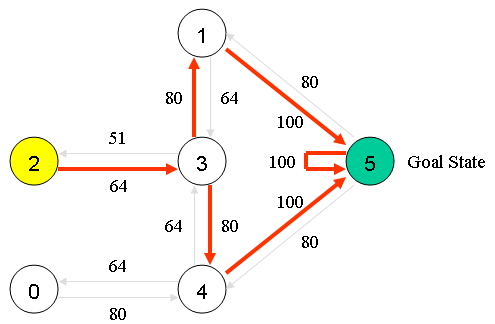```import numpy as np
# Q 矩阵初始化为0
q = np.matrix(np.zeros([6, 6]))
# Reward 矩阵为提前定义好的。 类似与HMM的生成矩阵。-1表示无相连接的边
r = np.matrix([[-1, -1, -1, -1,  0,  -1],
[-1, -1, -1,  0, -1, 100],
[-1, -1, -1,  0, -1,  -1],
[-1,  0,  0, -1,  0,  -1],
[ 0, -1, -1,  0, -1, 100],
[-1,  0, -1, -1,  0, 100]])

# hyperparameter
#折扣因子
gamma = 0.8
#是否选择最后策略的概率
epsilon = 0.4
# the main training loop
for episode in range(25):
print('first episode',episode)
# random initial state
state = np.random.randint(0, 6)
print('state',state)
# 如果不是最终转态
while (state != 5):
# 选择可能的动作
# Even in random case, we cannot choose actions whose r[state, action] = -1.
possible_actions = []
possible_q = []
for action in range(6):
if r[state, action] >= 0:
possible_actions.append(action)
possible_q.append(q[state, action])

# Step next state, here we use epsilon-greedy algorithm.
action = -1
if np.random.random() < epsilon:
# choose random action
action = possible_actions[np.random.randint(0, len(possible_actions))]
print('actionif',action)
else:
# greedy
action = possible_actions[np.argmax(possible_q)]
print('actionelse',action)

# Update Q value
q[state, action] = r[state, action] + gamma * q[action].max()

# Go to the next state
state = action

print("Training episode: %d" % episode)
print(q)
print("------------------------------------------------")
'''
# Display training progress
if episode % 10 == 0:
print("------------------------------------------------")
print("Training episode: %d" % episode)
print(q)
'''```